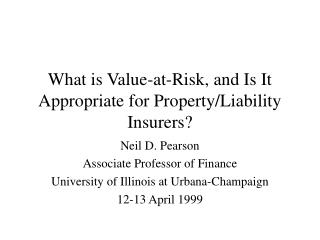Download PresentationWhat is Value-at-Risk, and Is It Appropriate for Property/Liability Insurers?

# What is Value-at-Risk, and Is It Appropriate for Property/Liability Insurers? - PowerPoint PPT PresentationDownload Presentation## What is Value-at-Risk, and Is It Appropriate for Property/Liability Insurers?

- - - - - - - - - - - - - - - - - - - - - - - - - - - E N D - - - - - - - - - - - - - - - - - - - - - - - - - - -
##### Presentation Transcript

1. What is Value-at-Risk, and Is It Appropriate for Property/Liability Insurers? Neil D. Pearson Associate Professor of Finance University of Illinois at Urbana-Champaign 12-13 April 1999

2. What is Value-at-Risk?

3. If You Like the Normal Distribution

4. What is Value-at-Risk? • Notation: DV change in portfolio value f(DV) density of DV x specified probability, e.g. 0.05 • Value-at-risk (VaR) satisfies or

5. What is Value-at-Risk? • Value-at-risk (for a probability of x percent) is the x percent critical value • If you like the normal distribution, it is proportional to the portfolio standard deviation

6. What is Value-at-Risk? • Value-at-risk is something you already understand • Value-at-risk is a particular way of summarizing the probability distribution of changes in portfolio value • The language of Value-at-Risk eases communication

7. If Value-at-Risk Isn’t New, Why Is It So Fashionable? • It provides some information about a firm’s risks • It is a simple, aggregate measure of risk • It is easy to understand • “Value” and “risk” are business words

8. Basic Value-at-Risk Methodologies • 3 methodologies: • Historical simulation • Variance-covariance/Delta-Normal/Analytic • Monte Carlo simulation • Illustrate these using a forward contract • current date is 20 May 1996 • in 91 days (19 August) • receive 10 million • pay \$15 million

9. First step: Identify market factors Market factors: S, rGBP, and rUSD

10. Historical Simulation • Start with current situation: • date: 20 May 1996 • portfolio: 1 forward contract • market factors: S=1.54, rGBP=6.06%, and rUSD=5.47% • Obtain values of market factors over last N days • Use changes in market factors to: • simulate values of market factors on 21 May • compute mark-to-market values of forward contract on 21 May • compute hypothetical profit/loss

11. Historical simulation: P/L

12. Repeat N times

13. Sort

14. Variance-covariance method

15. Portfolio standard deviation • Portfolio standard deviation • Portfolio variance Xi = dollar investment in i-th instrument si = standard deviation of returns of i-th instrument rij = correlation coefficient

16. Risk mapping: Main idea

17. Risk mapping: Main idea • The option price change resulting from a change in the oil price is: • In this sense the option “acts like” D barrels of oil • The option is “mapped” to D barrels of oil

18. Risk mapping: Interpret forward as portfolio of standardized positions • Change in m-t-m value of forward: • Find a portfolio of simpler (“standardized”) instruments that has same risk as the forward contract • “Same risk” means same factor sensitivities etc.

19. Risk mapping: Interpret forward as portfolio of standardized positions • Let V = X1 + X2 + X3 denote value of portfolio of standardized instruments • each standardized instrument depends on only 1 factor • Change in V is • Choose X1, X2, X3 so that:

20. Choice of X1, X2, X3 • Recall that the m-t-m value of the forward is • This implies

21. Compute variance of portfolio of standardized instruments • Variance of portfolio of standardized instruments: where and sUSD is the standard deviation of % changes in the \$ interest rate.

22. Compute value-at-risk • Portfolio standard deviation • Value-at-risk

23. Variance-covariance method

24. Monte Carlo simulation • Like historical simulation • Use psuedo-random changes in the factors rather than actual past changes • Psuedo-random changes in the factors are drawn from an assumed multivariate distribution

25. What Is VaR, Again • One need not focus on change in portfolio value over the next day, month, or quarter • Instead, one could estimate the distribution of: • cash flow • net income • surplus • or almost anything else one cares about • VaR (broadly defined)  DFA

26. Is VaR Appropriate for Property/Liability Insurers? • Do property/liability insurers have investment portfolios? • Do they care about the possible future values of things like: • Cash flow? • Net income? • Surplus?

27. What is Value-at-Risk?

28. Limitations of VaR • VaR  DFA • it is a particular, limited summary of the distribution • VaR is an estimate of the x percent critical value • based on various assumptions • sampling variation • VaR doesn’t indicate what circumstances will lead to the loss • 2 portfolios with opposite interest rate exposure could have same VaR